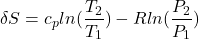# Enthalpy vs Entropy – Differences, H-S Chart, and Applications

#### Engineering

Enthalpy vs entropy -what’s the difference? All materials contain both of these thermodynamic properties. Enthalpy provides the total amount of internal energy held within a substance. Entropy measures the randomness in the material, also understood as the unavailability of energy, within the said compound. In this article, you will learn the differences between enthalpy and entropy, the H-S Chart, and applications of these properties in the real world.

## Differences of Enthalpy and Entropy

Where enthalpy is a measurement of energy potential, entropy measures the randomness of energy with relation to heat. Understanding enthalpy and entropy clarify what these differences mean and when to use each measurement.

### Enthalpy

Enthalpy represents the total energy within a substance, however, it is impossible to directly measure. Practically, it measures a change in total internal energy. This classifies the property as a non-physical property. It calculates as the combination of the internal energy(E) with pressure(P) and volume(V).Where internal energies relation is easy to see, the pressure and volume may not be as clear. The pressure and volume represent the work done by the material against the ambient pressure.

### Entropy

Entropy provides a statistic of microscopic uncertainty within a material, colloquially known as disorder. It relates to the heat capacity of the substance and other externally influenced properties such as temperature and pressure. Entropy derives from the total heat transfer(Q) and temperature(T).With heat capacity(Cp), initial and final Pressure(P), initial and final temperature(T), and the gas constant(R) provided, users may determine the change in entropy.Although entropy represents randomness, it can still be calculated and stay consistent with the trends.

## H-S Chart

Graphing enthalpy vs entropy allows for the determination of some key properties of the subject material. The lines on the chart provide all possible ranges of different values such as pressure, temperature, or even quality. The graph allows these property determinations from known entropy and enthalpy values. The inverse is also true as known physical value properties allow for the determination of enthalpy or entropy.

In this specific graph, there are three sets of colored lines going across the graph. The blue lines on the graph represent the pressure lines of the material. As enthalpy increases, so does the pressure, whereas as entropy increases the pressure decreases. Values read from the edge of the graph along the top and right sides.

The green line represents quality, however, the material is only able to exist as a liquid/vapor mixture underneath the dark line. As shown above, this dark line represents 100% quality which means that all of the material is not a vapor, thus quality cannot increase anymore. The red lines represent the temperature ranges of the material, which change exponentially as enthalpy increases, but only minutely as entropy increases.

The relations between the enthalpy, entropy, and other properties of a material may appear complicated, however, their relationship appears clear on the H-S chart. Thus, the H-S chart holds a useful purpose of only needing to know some values and being able to solve for the others.

## Applications of These Properties

Knowing when to use entropy vs. enthalpy allows engineers and system designers to more fully understand and apply thermodynamic cycles and thermal behavior. Both properties relate the temperature, pressure, and volume of a system in more robust terms.

### Applications of Entropy

Entropy’s most common application occurs in the heat transfer process. The combustion engine provides a common example of keeping entropy manipulation constant to find other properties. Simple applications like boiling water on a stove may use entropy to determine other properties.